# RECLASSIFICATION

The reclassification (or, simply, classification) operator, proposed in Rafanelli & Ricci (1984, 1985), and subsequently in Fortunato et al. (1986) and in Rafanelli & Ricci (1993), substitutes a set (or possibly all) of the category attributes in a MAD, with another set, according to the relation existing between two sets of category attributes. This fact means that there is a redefinition of the data in the MAD, with a computation of the summary values (for this reason it is often referred to by the term redefine). This operation classifies one category attribute of a MAD according to a given relation in which the new classification criteria are specified. For example, the category attribute "months" can be classified in "quarter" according to the relation "{January, February, March}" "1st quarter," etc. This operator is also able to reclassify a set (or possibly all) of the category attributes of the MAD with another set of attributes, according to a given relation, by means of a substitution. The schema of this relation contains both the category attributes which must be substituted, and the category attributes which must substitute the above-mentioned attributes. The resulting MAD is a redefinition of the initial data, with (in general) an aggregation of data (recalculation of the summary values).

Let us consider, for example, the MAD "number_of_ cars_produced_in_Japan" of Figure 16(a), characterized by the category attributes "model" and "years"; if we wish to have the same MAD described by the category attributes "piston displacement" and "years" (the link between "model and "piston displacement" is the relation "r" in Figure 16(b)), we perform the classification of "number_of_ cars_produced_in_Japan" by the relation "r" along the category attributes "piston displacement" and "years," and we obtain the MAD of Figure 16(c).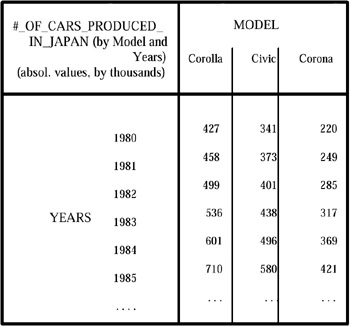Figure 16a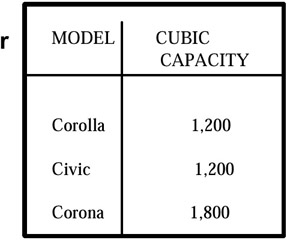Figure 16b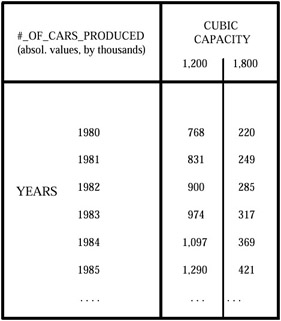Figure 16c

This operator is more powerful than the roll-up operator (discussed in the following), because it allows aggregation only along a defined hierarchy, while the reclassification operator also allows another grouping of the lower level of the hierarchy to be defined ex-novo, giving a grouping relation not defined a priori, but proposed by the user. Generally, it changes the cardinality of the MADs, decreasing them according to a functional dependency defined by the relation mentioned above.

Analogous to the previous operators, let Rx be the set of all the relations (of the micro database) involved in the production of all the MADs which describe a given phenomenon; we considered the subset of Rx formed only by the relations involved in the building of fact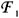. As already explained, we call this subset an aggregation relation, and denote it by the expression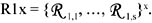. Let {A1j} be the set of category attributes which define the descriptive space of the MAD s1. Let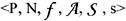be the six-tuple which defines s1. Let {Ah} be a set of attributes, different from the set of category attributes which form A, such that a functional dependency between a subset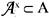and {Ah} exists. We denote this functional dependency by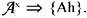. Then, the reclassification of s1 respective to the functional dependency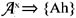produces a new MAD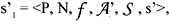where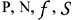are the same and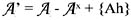s' is a set of recomputed summary values. This recomputation depends on the type of aggregation function (e.g., count, sum, average) applied to the original microdata.

Obviously, the new MAD s'1 is a more aggregate representation of the fact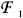described by the MAD s1. Moreover, in general the number of dimensions of s'1 is less than s1, i.e., its cardinality is smaller. It is important to note that part of the dimension changes, so that also the descriptive space changes. In the case of Figure 16, the dimension number remains the same, but the descriptive space changes from <Model, Year> to <Model, Cubic capacity>.Multidimensional Databases: Problems and Solutions
ISBN: 1591400538
EAN: 2147483647
Year: 2003
Pages: 150